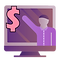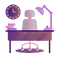top of page## Counterparty Risk, Artificial Intelligence and Quantum Algorithms

COURSE OBJECTIVE

Course on counterparty risk modeling in a financial institution that covers the following objectives:

• Explain the recent Basel III directives on counterparty risk default capital charge, IMM and standard approaches

• As well as the recent Basel III directives for the risk capital charge of Credit Value Adjustment CVA under the basic and standard approach.

• Recent methodologies to calculate the XVA and the necessary adjustments in the pricing of Over The Counter OTC derivatives related to counterparty risk, financing, collateral and capital are exposed.

• Models are explained to calculate the Debit Value Adjustment DVA, and other adjustments such as LVA, FVA, CollVA, KVA and XVA.

• Methodologies are shown to estimate the parameters used in the CVA such as the probability of default PD, severity of loss LGD and Credit Spread using structural models and reduced form models.

• Explain the modeling of current exposure and the main metrics used such as potential future exposure and expected exposure.

• Present methodologies for calculating the Wrong Way Risk WWR

• Evaluate some of the most used derivatives in banking.

• Present counterparty risk validation techniques for CVA and Expected Exposure.

• Explain CVA and XVA counterparty risk stress testing model.​​

• ARTIFICIAL INTELLIGENCE

• Show traditional and innovative artificial intelligence methodologies such as Deep Learning and machine learning to value derivatives, estimate exposures, calculate CVA and XVA.

​​

• QUANTUM ALGORITHMS and MACHINE LEARNING

• Quantum mechanics is well known for speeding up statistical sampling processes over classical techniques. In quantitative finance, statistical sampling comes up in many use cases.

• Quantitative algorithms for the calculation of the Credit Value Adjustment (CVA) are explained, and we expose opportunities and challenges of the quantum advantage.

• We address how to obtain a quantum advantage over the Monte Carlo simulation in the pricing of derivatives.

• We explain numerical analyzes to show the quantum acceleration, with respect to economic capital, on classical Monte Carlo simulations.

• Quantum machine learning explained.

• Use of tensor networks to improve the speed of neural networks.

WHO SHOULD ATTEND?

This program is aimed at directors, managers, consultants, regulators, auditors and counterparty credit risk analysts, as well as those professionals who are implementing the Basel III regulatory agreements. Professionals who work in banks, savings banks and all those companies that are exposed to credit risk. It is important to have knowledge of Statistics and Probability as well as Excel.Price: 9.900 €

Schedules:

• Europe: Mon-Fri, CEST 16-20 h

• America: Mon-Fri, CDT 18-21 h

• Asia: Mon-Fri, IST 18-21 hDuration: 40 hMaterial:

• Presentations PDF

• Exercises in Excel, R , Python, Jupyterlab y Tensorflow## Counterparty Risk, Artificial Intelligence and Quantum Machine LearningAnchor 10

Modular Agenda

Quantum Computing and Artificial Intelligence

​Module -1: Quantum Computing and Algorithms (Optional)

• Future of quantum computing in banking

• Is it necessary to know quantum mechanics?

• QIS Hardware and Apps

• quantum operations

• Qubit representation

• Measurement

• Overlap

• matrix multiplication

• Qubit operations

• Multiple Quantum Circuits

• Entanglement

• Deutsch Algorithm

• Quantum Fourier transform and search algorithms

• Hybrid quantum-classical algorithms

• Quantum annealing, simulation and optimization of algorithms

• Quantum machine learning algorithms

• Exercise 1: Quantum operations

Module 0: Deep Learning for Exhibition (Optional)

​​

• Definition and concept of deep learning

• Why now the use of deep learning?

• Neural network architectures

• activation function

• sigmoidal

• Rectified linear unit

• hypertangent

• Softmax

• Multilayer Perceptron

• Using Tensorflow

• Using Tensorboard

• R deep learning

• Python deep learning

• Typology of Neural Networks

• feedforward network

• Convolutional Neural Networks CNN

• Recurrent Neural Networks RNN

• Use of deep learning in banking

• cost function

• Use of deep learning for the IRRBB and ALM

• Deep Learning Software​

• Deployment software: Nvidia and Cuda

• Hardware, CPU, GPU and cloud environments

• Deep Learning for valuation of derivatives

• Stochastic Differential Equations

• Optimization models